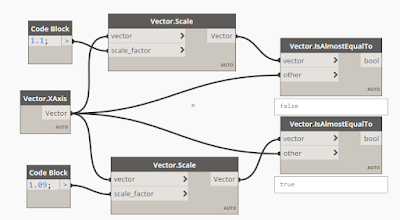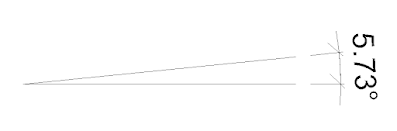## Wednesday, July 21, 2021

### What is Vector.AlmostEqualTo and Vector.IsParallel?

Vector is one of the most important factors when you manipulate geometries with Dynamo.

If so, Here is a question.What will these two return???

As known, a vector has two factors in it. One is Direction, another is Length.

I wondered what does 'Almost' means In Vector.AlmostEqualTo node. There is no 'Almost' kind of things in Math. There are only Right or Wrongs.

So, I've tested the node with some vectors which are Almost Equal (for me) to each other to find out what 'Almost' means in the node.

1. Almost Equal LengthAs you can see above, 1 and 1.09 are almost equal lengths. But 1 and 1.1 are totally different lengths. It means under 10% longer lengths are regarded as Almost Equal.

2. Almost Equal Direction

According to the test, under 5.74 degree difference is Almost Equal and others are totally different direction for the node. And of course 180 degree difference is also totally different direction.Almost Equal Direction by the node

So, if you want to take 'Almost Equal Direction' while ignoring Lengths of both, you can use Vector.Normalized to make both Lengths to the same.

3. Parallel

I don't think I have to explain 'Parallel', but I tested Vector.IsParallel node to make it sure.
Only the same direction and 180 degree rotated direction are regarded as Parallel. Lengths of both vectors are not considered.

4. After above experiments were conducted, a simple Python code for Dynamo named "Vector.IsAlmostParallel" can be wrote.

You can use below code if necessary.

Enjoy :)

```# Enable Python support and load DesignScript library
import clr
from Autodesk.DesignScript.Geometry import *

# The inputs to this node will be stored as a list in the IN variables.
dataEnteringNode = IN

# Place your code below this line
vector1=IN
vector2=IN

v1 = vector1.Normalized()
v2 = vector2.Normalized()
v3 = v2.Reverse()

# determine Almost Equal Angle Only.
if v1.IsAlmostEqualTo(v2):
IsAlmostEqualDirection=True
else:
IsAlmostEqualDirection=False

# determine Almost Parallel.
if v1.IsAlmostEqualTo(v2) or v1.IsAlmostEqualTo(v3):
IsAlmostParallel=True
else:
IsAlmostParallel=False

# Assign your output to the OUT variable.
# Use IsAlmostEqualDirection if you want.
OUT = IsAlmostParallel
```